C Program to Calculate Purchase Amount to be Paid after Discount

In this tutorial you will learn about the C Program to Calculate Purchase Amount to be Paid after Discount and its application with practical example.

C Program to Calculate Purchase Amount to be Paid after Discount

In this tutorial, we will learn to create a C program that will Purchase Amounts to be Paid after Discount using C programming.

Prerequisites

Before starting with the tutorial we assume that you are best aware of the following C programming topics:

• Operators in C Programming.
• Basic Input and Output function in C Programming.
• Basic C programming.
• Conditional statement in C programming.

Program to Calculate Purchase Amount to Pay after Discount

In c programming, it is possible to take numerical input from the user and Purchase Amounts to Pay after Discount with the help of a very small amount of code. The C language has many types of header libraries which has supported function in them with the help of these files the programming is easy.

Algorithm:-

With the help of this program, we can Purchase Amounts to Pay after Discount.

Output:-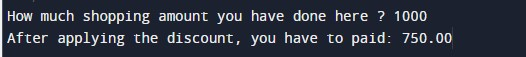In the above program, we have first initialized the required variable.

• amt = it will hold the float value for the basic amount.
• discount = it will hold the float value for the discount amount.
• amtToBePaid = it will hold the float value for the gross amount.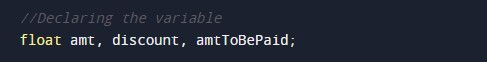Input amount from the user.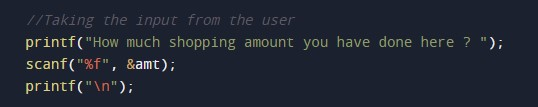Calculating the discount.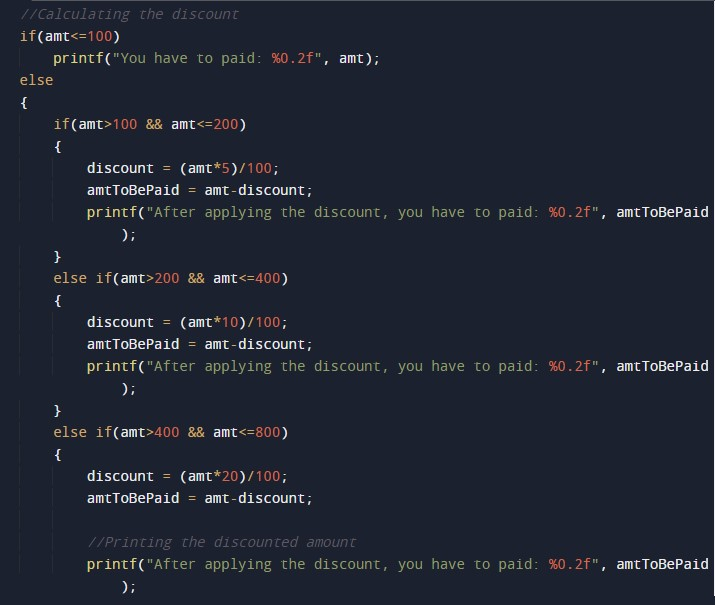Printing amount after giving the discount.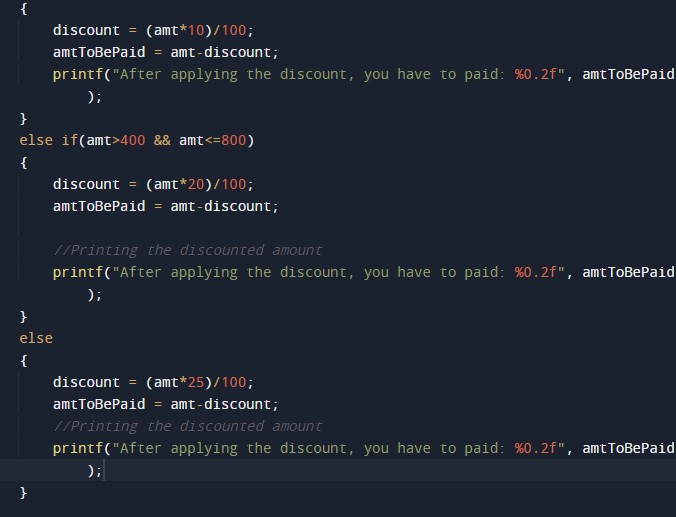In this tutorial we have learn about the C Program to Calculate Purchase Amount to be Paid after Discount and its application with practical example. I hope you will like this tutorial.